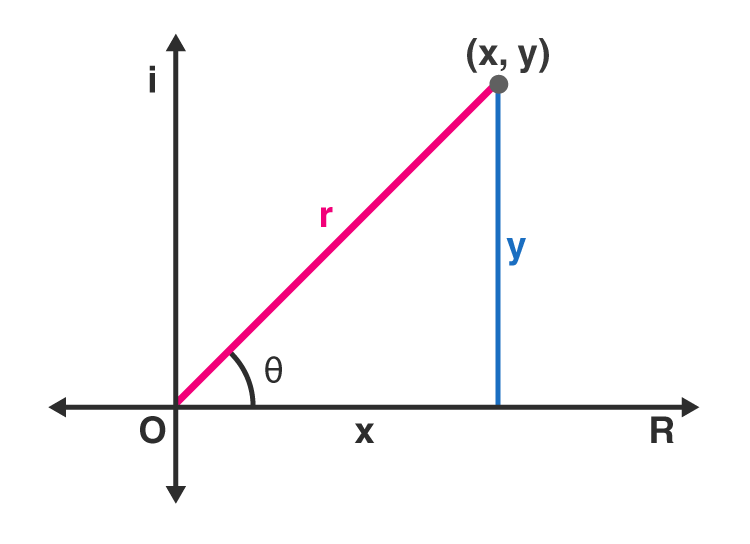# Polar Form of Complex Numbers

The polar form of a complex number is a different way to represent a complex number apart from rectangular form. Usually, we represent the complex numbers, in the form of z = x+iy where ‘i’ the imaginary number. But in polar form, the complex numbers are represented as the combination of modulus and argument.

The modulus of a complex number is also called absolute value. This polar form is represented with the help of polar coordinates of real and imaginary numbers in the coordinate system. Let us learn here, in this article, how to derive the polar form of complex numbers.

## Polar Form Formula of Complex Numbers

Let us consider (x, y) are the coordinates of complex numbers x+iy. Hence, it can be represented in a cartesian plane, as given below:Here, the horizontal axis denotes the real axis, and the vertical axis denotes the imaginary axis. The real and complex components of coordinates are found in terms of r and θ where r is the length of the vector, and θ is the angle made with the real axis.

Using Pythagorean Theorem, we can write;

r2 = x2 + y2

From trigonometric ratios, we know that;

Cos θ = Adjacent side of the angle θ/Hypotenuse

Cos θ = x/r

Also, sin θ = Opposite side of the angle θ/Hypotenuse

Sin θ =y/r

Multiplying each side by r :

rcosθ=x and rsinθ=y

The rectangular form of a complex number is denoted by:

z = x+iy

Substitute the values of x and y.

z = x+iy

= r (cosθ + i rsinθ)

In the case of a complex number, r signifies the absolute value or modulus and the angle θ is known as the argument of the complex number.

### Polar Form Equation

The equation of polar form of a complex number z = x+iy is:

z=r(cosθ+isinθ)

where

r=|z|=√(x2+y2)

x=r cosθ

y=r sinθ

θ=tan-1(y/x) for x>0

θ=tan-1(y/x)+π or

θ=tan-1(y/x)+180° for x<0 .

## Converting Rectangular form into Polar form

Let us see some examples of conversion of the rectangular form of complex numbers into polar form.

Example: Find the polar form of complex number 7-5i.

Solution:7-5i is the rectangular form of a complex number.

To convert into polar form modulus and argument of the given complex number, i.e. r and θ.

We know, the modulus or absolute value of the complex number is given by:

r=|z|=√x2+y2

r=√(72+(-5)2

r=√49+25

r=√74

r=8.6

To find the argument of a complex number, we need to check the condition first, such as:

Here x>0, therefore, we will use the formula,

θ=tan-1(b/a) = θ=tan-1(5/7) = 35.54°

Since 7−5i is in the fourth quadrant, so

θ=360°−35.54° = 324.46°

Hence, the polar form of 7-5i is represented by:

7−5i=8.6(cos 324.5°+i sin 324.5°)

## Adding Complex numbers in Polar Form

Suppose we have two complex numbers, one in a rectangular form and one in polar form. Now, we need to add these two numbers and represent in the polar form again.

Let 3+5i, and 7∠50° are the two complex numbers.

First, we will convert 7∠50° into a rectangular form.

7∠50° = x+iy

Hence,

x = 7 cos 50° = 4.5

y = 7 sin 50° = 5.36

So,

7∠50° = 4.5 + i 5.36

Therefore, if we add the two given complex numbers, we get;

(3+i5)+ (4.5 + i 5.36) = 7.5+i10.36

Again, to convert the resulting complex number in polar form, we need to find the modulus and argument of the number. Hence,

Modulus is equal to;

r=|z|=√(x2+y2)

r =√(7.52+10.362)

r=12.79

And the argument is equal to;

θ=tan-1(y/x)

θ=tan-1(10.36/7.5)

θ=54.1°

Therefore, the required complex number is 12.79∠54.1°.